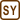## Refrigerating Engine No. 2

### Task number: 1804

An ideal refrigerating engine operating according to Carnot cycle transfers heat from a cold reservoir filled with water with a temperature of 0 °C to a hot reservoir filled with water with temperature of 100 °C. How much water needs to freeze in the cold reservoir, so that 1 kg of water in the hot reservoir evaporates and the temperature of the vapour is 100 °C?

• #### Hint

Start with the fact that the heat supplied Qsup to water in the hot reservoir is equal to the sum of the heat Qrel released by water in the cold reservoir and the work W performed during the transfer of the heat from the cold reservoir to the hot reservoir . Therefore, we can write the following equation

$Q_\mathrm{{sup}}=Q_\mathrm{{rel}}+W.$

Do you know how to express these unknowns using given quantities?

• #### Given values

 t1 = 0 °C => T1 = 273.15 K water temperature in the cold reservoir t2 = 100 °C => T2 = 373.15 K water temperature in the hot reservoir m2 = 1 kg mass of water in the hot reservoir m1 = ? mass of water in the cold reservoir

From The Handbook of Chemistry and Physics:

 lf = 334 kJ kg−1 = 334·103 J kg−1 specific latent heat of fusion of water le = 2256 kJ kg−1 = 2256·103 J kg−1 specific latent heat of evaporation of water
• #### Analysis

We start with the fact that the heat Qsup supplied to water in the hot sink is equal to the sum of the heat Qrel released by water in the cold reservoir and the work W performed during the transfer of the heat from the cold reservoir to the hot reservoir. We express the mentioned quantities using the given quantities.

The performed work is equal to the efficiency of the Carnot engine times the released heat. The efficiency of a Carnot engine depends only on the temperatures of the cold and hot reservoir.

The heat supplied to the evaporating water is defined as the product of the mass of water in the hot reservoir and its specific latent heat of evaporation.

Heat released by water in the cold reservoir is analogously defined as the product of the mass of water in the cold reservoir and its specific latent heat of fusion.

• #### Solution

The heat Qsup supplied to water in the hot reservoir is equal to the sum of heat Qrel released from water in the cold reservoir and the work W performed during the heat transfer from the cold to the hot reservoir:

$Q_\mathrm{{sup}}=Q_\mathrm{{rel}}+W.$

The work W can be expressed using the efficiency η and the released heat Qrel

$W=\eta Q_\mathrm{{rel}},$

where η is the efficiency of Carnot engine. The efficiency of ideal Carnot refrigerating engine is defined a follows:

$\eta = \frac{T_2-T_1}{T_1},$

where T1 is the temperature of water in the cold reservoir and T2 is the temperature of the water in the hot reservoir.

By substituting into the original equation, we obtain:

$Q_\mathrm{{sup}}=Q_\mathrm{{rel}}+W= Q_\mathrm{{rel}}+\eta Q_{rel} = \left(1+\frac{T_2-T_1}{T_1}\right)Q_\mathrm{{rel}}$

After adjusting we obtain

$Q_\mathrm{{sup}}= \frac{T_2}{T_1}Q_\mathrm{{rel}}.$

The heat supplied to the evaporating water is described using the definition of specific latent heat of evaporation le

$Q_\mathrm{{sup}}=m_2l_\mathrm{e},$

where m2 is the mass of water in the hot reservoir.

For the released heat it applies

$Q_\mathrm{{rel}}=m_1l_\mathrm{f},$

where m1 is the mass of water in the cold reservoir and lf is the specific latent heat of fusion of water.

After substituting the above mentioned equations we obtain

$m_2l_\mathrm{e}= \frac{T_2}{T_1}m_1l_\mathrm{f}.$

After adjusting the expression we get the final relation for the unknown mass m1 of water in the cold reservoir

$m_1=\frac{m_2l_\mathrm{e}T_1}{T_2l_\mathrm{f}}.$
• #### Numerical solution

$m_1=\frac{m_2l_\mathrm{e}T_1}{T_2l_\mathrm{f}}$ $m_1= \frac{1\cdot{ 2256}\cdot{ 10^3}\cdot273.15}{373.15\cdot {334}\cdot{ 10^3}}\,\mathrm{kg}\,\dot{=}\,5\,\mathrm{kg}$
• #### Answer

It is necessary to freeze about 5 kg of water in the cold reservoir.